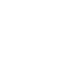Analysis Services

# Multiple Time Utility Dimensions

Time Utility Dimensions (or, strictly speaking with AS2005 attribute hierarchies) are useful things. I first learnt about them from George Spofford through various newsgroup postings and the first version of ‘MDX Solutions’ and they have become one of the most commonly used design techniques in Analysis Services cubes; indeed, they have been built into the product in the form of the ‘Define Time Intelligence’ functionality of the ‘Add Business Intelligence’ wizard.

So what is there that’s new to say about them? Well, sometimes it’s useful to have more than one. Take for example the following bit of MDX Script created by the wizard that creates two calculated members (Year-To-Date and Year-Over-Year Growth) on the [Year-Month Period Calculations] attribute on a simple time dimension:

/*

Begin Time Intelligence script for the [Period].[Year-Month] hierarchy.

*/

Create Member

CurrentCube.[Period].[Year-Month Period Calculations].[Year to Date]

As "NA" ;

Create Member

CurrentCube.[Period].[Year-Month Period Calculations].[Year Over Year Growth]

As "NA" ;

Scope(

{

[Measures].[Sales]

}

) ;

// Year to Date

(

[Period].[Year-Month Period Calculations].[Year to Date],

[Period].[Year].[Year].Members,

[Period].[Month].Members

) =

Aggregate(

{ [Period].[Year-Month Period Calculations].DefaultMember } *

PeriodsToDate(

[Period].[Year-Month].[Year],

[Period].[Year-Month].CurrentMember

)

) ;

// Year Over Year Growth

(

[Period].[Year-Month Period Calculations].[Year Over Year Growth],

[Period].[Year].[Year].Members ( 1 ) : Null,

[Period].[Month].Members

) =

( [Period].[Year-Month Period Calculations].DefaultMember ) –

( [Period].[Year-Month Period Calculations].DefaultMember,

ParallelPeriod(

[Period].[Year-Month].[Year],

1,

[Period].[Year-Month].CurrentMember

)

) ;

(

[Period].[Year-Month Period Calculations].[Year Over Year Growth],

[Period].[Year].[Year].Members ( 0 ),

[Period].[Month].Members

) = Null ;

End Scope ;

/*

End Time Intelligence script for the [Period].[Year-Month] hierarchy.

*/

This works fine, but what happens when your users ask to be able to look at Year-Over-Year Growth for the Year-To-Date values? Of course you could create a third calculated member on the hierarchy which implemented this, but you can probably guess where I’m going with this: the more combinations of calculations that you need, the greater the number of calculated members you need to create and maintain.

Here’s where the value of multiple time utility attribute hierarchies comes in because they can help you control this explosion of calculated members – you can make them work together instead. To do this, first you need to create a new attribute hierarchy on your time dimension by dragging and dropping the same column as was used to create your original time utility attribute hierarchy; you need to make sure to set its IsAggregatable property to false so that it doesn’t have an All Member. Then you need to decide which calculations should go on which hierarchy, which of course dictates which combinations of calculations you have available. Here’s the MDX Script example from earlier rewritten so that the two calculated members are now on two different attribute hierarchies called [Period].[Year-Month Period Calculations] and [Period].[Year-Month Period Calculations2]:

/*

Begin Time Intelligence script for the [Period].[Year-Month] hierarchy.

*/

Create Member

CurrentCube.[Period].[Year-Month Period Calculations].[Year to Date]

As "NA" ;

Create Member

CurrentCube.[Period].[Year-Month Period Calculations 2].[Year Over Year Growth]

As "NA" ;

Scope(

{

[Measures].[Sales]

}

) ;

// Year to Date

(

[Period].[Year-Month Period Calculations].[Year to Date],

[Period].[Year].[Year].Members,

[Period].[Month].Members

) =

Aggregate(

{ [Period].[Year-Month Period Calculations].DefaultMember } *

PeriodsToDate(

[Period].[Year-Month].[Year],

[Period].[Year-Month].CurrentMember

)

) ;

// Year Over Year Growth

(

[Period].[Year-Month Period Calculations 2].[Year Over Year Growth],

[Period].[Year].[Year].Members ( 1 ) : Null,

[Period].[Month].Members

) =

( [Period].[Year-Month Period Calculations 2].DefaultMember ) –

( [Period].[Year-Month Period Calculations 2].DefaultMember,

ParallelPeriod(

[Period].[Year-Month].[Year],

1,

[Period].[Year-Month].CurrentMember

)

) ;

(

[Period].[Year-Month Period Calculations 2].[Year Over Year Growth],

[Period].[Year].[Year].Members ( 0 ),

[Period].[Month].Members

) = Null ;

End Scope ;

/*

End Time Intelligence script for the [Period].[Year-Month] hierarchy.

*/

Now the user can crossjoin the two calculated members in their queries to get the Year-Over-Year Growth for the Year-To-Date, as well as use them separately.

In practice it’s actually quite tricky to determine which calculated members should go on each hierarchy and deal with the associated problem of solve order: for example if we added a 12 Month Moving Average calculation to this script, would we want to see the average of the Year-Over-Year growth or the growth of the average, or both or neither? There’s also another downside to this approach which is that if users found it hard to understand the concept of one time utility attribute hierarchy then they are guaranteed to be confused when they have more than one, though this should be balanced against the usability and maintainability problems inherent in having more than about ten combination-style calculated members on one attribute hierarchy. Overall, I’ve used this technique three or four times now (once even using three separate hierarchies) and it’s proved to be very successful when either the users have been very sophisticated or when the cube is only queried via Reporting Services.

## 4 thoughts on “Multiple Time Utility Dimensions”

1.anchky says:

may i translate this article into chinese and post it to http://www.cnblogs.com?

2.Chris says:

Yes, no problem so long as you include a link back here and clearly state this blog as the source.